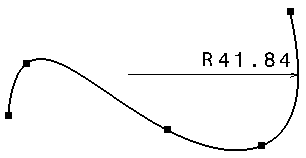This task will show you how to create and modify a curvature radius dimension. A curvature radius dimension lets you know the curvature radius at a given point on a curve (spline, ellipse, etc.).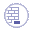Create a spline.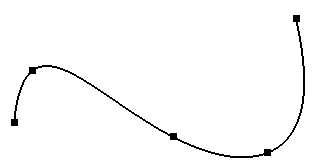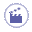1. Select Dimension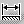in the Dimensioning toolbar.

2. Move the cursor over the spline. You can notice that the cursor changes to indicate that you are going to create a dimension on a spline.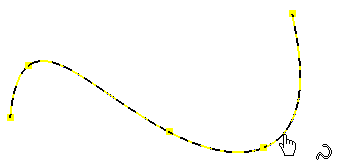3. On the spline, click the point where you want to create the curvature radius dimension. A preview of the curvature radius dimension is displayed.

4. Click to validate the dimension creation.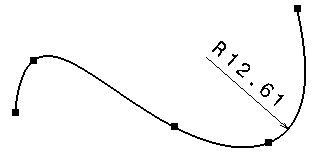5. Move the dimension over the spline to modify the dimension.